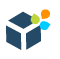DWBI.org Login / Sign Up
New Account?
Recovery
SAP Data Services

# Data Services Scenario Questions Part 1

Consider the following Source data in a flat file:

 DEPTNO SALARY 10 1000 20 2000 30 3000 40 4000

Scenario 1: Let's try to load the Cumulative Sum of salaries of the departments into the target table. The target table data should look like below:

 DEPTNO SALARY CUMULATIVE_SALARY 10 1000 1000 20 2000 3000 30 3000 6000 40 4000 10000

#### Solution:

1. Let us first define the Source File Format. This same file format will be reused for the next set of the scenario questions.

2. Next we create a new Batch Job, say JB_SCENARIO_DS. Within the Job we create a Data Flow, say DF_SCENARIO_1.

3. At the Data flow level i.e. Context DF_SCENARIO_1, we Insert a new Parameter using the Definitions tab. Let's name it as \$PREV_SAL with Data type decimal(10,2) and Parameter type as Input.

At the Job level i.e. Context JB_SCENARIO_1, we initialize the Parameter \$PREV_SAL using the Calls tab. We set the Argument value to 0.00

4. Next we create a New Custom Function from the Local Object Library. Let's name it CF_CUME_SUM_SAL.

Within the Custom Function Smart Editor, first we Insert two Parameters, namely \$CURR_SAL and \$PREV_SAL with Data type decimal(10,2) with Parameter type as Input and Input/Output respectively.

Also we modify the Return Parameter Data type to decimal(10,2).

5. Next we define the custom function as below and Validate the same.

``````\$PREV_SAL = \$CURR_SAL + \$PREV_SAL;

Return \$PREV_SAL;``````

The purpose of defining the Parameter and Custom Function is to perform Parameter Short-circuiting. Here within the function, we basically set the \$PREV_SAL Parameter of type Input/Output to sum of salaries till the current processing row. Since it is of type Input/Output the calculated sum value or the retained sum of salary is passed back into the Dataflow Parameter. So by using Custom Function we can modify and pass values to a Dataflow Parameter. Hence the Parameter defined at Dataflow level is short-circuited with the Input/Output Parameter of the Custom Function.

6. Let's go back and design the Data flow. First of all we take the File Format defined earlier, from the Local Object Library as Source.

7. Next we place a Query transform, say QRY_CUME_SUM. First we select the columns DEPTNO and SALARY from the Schema In of the Query transform and Map to Output.

Next we specify a New Function Call in Schema Out of the Query transform. Choose the Custom Functions from the Function categories and select the Function name CF_CUME_SUM_SAL.

Next we Define Input Parameters. We specify the inputs as below:

``````\$CURR_SAL = FF_SRC_DEPT.SALARY

\$PREV_SAL = \$PREV_SAL``````